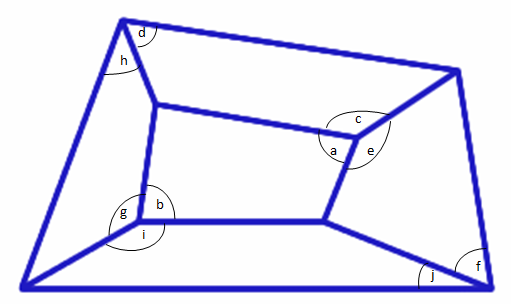#### You may also likeFour rods are hinged at their ends to form a convex quadrilateral. Investigate the different shapes that the quadrilateral can take. Be patient this problem may be slow to load.### Lawnmower

A kite shaped lawn consists of an equilateral triangle ABC of side 130 feet and an isosceles triangle BCD in which BD and CD are of length 169 feet. A gardener has a motor mower which cuts strips of grass exactly one foot wide and wishes to cut the entire lawn in parallel strips. What is the minimum number of strips the gardener must mow?### Long Short

What can you say about the lengths of the sides of a quadrilateral whose vertices are on a unit circle?

##### Age 14 to 16 Challenge Level:

We had two very clear solutions to this problem - well done to James, from Poole Grammar School, and Dylan, who did not give his school. James's solution is shown here.All five of the small quadrilaterals in the above shape are cyclic, and by using the fact that opposite angles in a cyclic quadrilateral add up to $180 ^{\circ}$ we can prove that the large quadrilateral made up of the five smaller ones is also cyclic.

Opposite angles in each cyclic quadrilateral add up to $180^{\circ}$, so we can write expressions for angles $a$, $c$, and $e$.

$$a = 180^{\circ}- b$$ $$c = 180^{\circ}- d$$ $$e = 180^{\circ}- f$$

Angles $a$, $c$, and $e$ are all round the same point therefore: $$360^{\circ} = a + c + e$$ Substituting in the expressions for angles c and e in we get: $$360^{\circ} = a + (180^{\circ} - d) + (180^{\circ} - f)$$

Simplifying this we get: $$360^{\circ} = a + 360^{\circ} - d - f$$ $$a = d + f$$

Now we follow exactly the same working with the other side of the quadrilateral which gives us the equation $$b = h + j$$

We also know that $a + b = 180^{\circ}$ because they are opposite angles in a cyclic quadrilateral. Substituting in the expressions for $a$ and $b$ gives us $$d + f + h + j = 180^{\circ}$$
So $$(d + h) = 180^{\circ} - (f + j)$$

Looking back at the original diagram we can see that $(d + h)$ and $(f +j)$ are opposite angles in the quadrilateral and because $(d + h) = 180^{\circ} - (f + j)$ the quadrilateral must be cyclic.